# A Reference Design for High-Performance, Low-Cost Weigh Scales

### Introduction

The trend in weigh scales towards higher accuracy and lower cost has produced an increased demand for high-performance analog signal processing at low cost. The scope of this requirement is not obvious; most weigh scales output the final weight value at a resolution of 1:3,000 or 1:10,000, which is easily met (apparently) by a 12-bit to 14-bit ADC (analog-to-digital converter). However, a closer examination of weigh scales shows that meeting the resolution requirement is not that easily accomplished; in fact, the ADC accuracy needs to be closer to 20 bits. In this article, we discuss some of the system specifications of weigh scales and deal with considerations for designing and building a weigh-scale system. The main areas considered are peak-to-peak-noise resolution, A/D-converter dynamic range, gain drift, and filtering. We compare measured data from a real load cell to inputs from a stable voltage reference, using a weigh-scale reference design as an evaluation board.

### Load-Cell Sensor

The most common weigh-scale implementation is to use a bridge-type load-cell sensor, with voltage output directly proportional to the weight placed on it. A typical load-cell bridge is illustrated in Figure 1; it is a 4-resistor bridge circuit with at least two variable arms, where the resistance change with weight applied creates a differential voltage at a common-mode level of 2.5 V (one-half the supply voltage). A typical bridge will have resistors of the order of 300 ohms.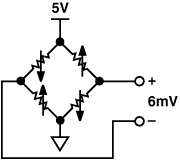Figure 1. Basic circuit of load cell.

The load cell is inherently monotonic. The main parameters of the load cell are sensitivity, total error, and drift.

#### Sensitivity

A typical load cell’s electrical sensitivity, defined as the ratio of the full-load output to the excitation voltage, is 2 mV/V. With 2-mV/V sensitivity and 5-V excitation, the full-scale output voltage is 10 mV. Often, in order to use the most linear portion of the load cell’s span, only about two-thirds of this range would be used. The full scale output voltage would thus be about 6 mV. The challenge thus posed is to measure small signal changes within this 6-mV full-scale range in such a way as to get the highest achievable performance—not an easy task in the industrial environments where weigh scales would typically be used.

#### Total Error

The total error is the ratio of the output error to the rated output. A typical weigh scale has a total error specification of about 0.02%. It is a very important specification, because it limits the accuracy that could be reached with an ideal signal conditioning circuit. It thus determines the choice of A/D-converter resolution, as well as the design of the amplification circuit and filter.

#### Drift

Load cells also drift over time. Figure 2 shows an actual load-cell drift characteristic, measured over a 24-hour period. Temperature was essentially constant during the measurement period, so the drift is not temperature-related. The result shown (number of bits change, measured using a 24-bit ADC), shows a total drift of 125 LSBs, or about 7.5 ppm.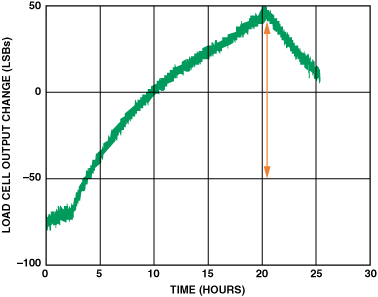Figure 2. Long-term load-cell stability—24-hour plot.

### Weigh-Scale System

The most important parameters to consider when designing a weigh-scale system are internal count, ADC dynamic range, noise-free resolution, update rate, system gain, and gain-error drift. The system must be designed to be ratiometric, hence independent of supply voltage—this will be discussed later.

#### Internal Count

As mentioned, the resolutions of typical weigh-scale systems, as seen by the user, range from a count of 1:3,000 at the low end up to 1:10,000 for high-end solutions. For example, a weigh scale that can measure up to 5 kilograms with a count of 1:10,000 has a weight resolution of 0.5 grams. This resolution, as seen on the LCD display, is generally referred to as the external count. In order to guarantee that this resolution is met accurately, the internal resolution of the system must be better by at least an order of magnitude. In fact, some standards dictate that the internal count of the system be a factor of 20 times better than that of the external count. For the example above, the internal count would need to be 1:200,000.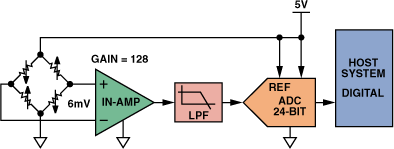Figure 3. Typical weigh-scale system.

#### ADC Dynamic Range

In weigh-scale applications using standard high-resolution A/D converters, the entire full-scale range of the ADC is unlikely to be used. In the example of Figure 1, the load cell has a 5-V supply and a full-scale output of 10 mV. The linear range is 6 mV. Using a gain-of-128 stage on the front end, the ADC input will see about 768 mV full-scale. If a standard 2.5-V reference is used, only 30% of the ADC’s dynamic range is used.

If the internal count needs to be 1:200,000 accurate for the full-scale range of 770 mV, the ADC therefore needs to be of the order of 3× to 4× better in order to meet the performance requirements. In this case, for a count of 1:800,000, the ADC would require 19 bits to 20 bits of accuracy. The practical challenge posed by the signal-processing requirement can now be understood.

#### Gain and Offset Drift

Industrial weigh-scale systems typically operate over a 50-degree (Celsius) temperature range. Designers must consider the accuracy of the system at temperatures beyond room temperature, since gain drift with temperature can be a dominant source of error. For example, a 20-bit stable system with a 1-ppm/°C gain-error drift will have 50 LSBs of error over a 50-degree range. Even though the system may be 1-LSB stable at 25°C, it is in effect only 50-LSBs accurate over the full temperature range. Choosing an ADC with low gain drift is thus a very important consideration when designing weigh scales.

Offset drift is not as big a consideration. Most sigma-delta ADCs are designed with inherent chopping-mode techniques, which give the advantage of lower drift and better immunity to 1/f noise—useful features for weigh-scale designers. For example, the AD7799 A/D converter has an offset drift specification of 10 nV/°C. In a 20-bit system, this would contribute a total of only 1/4-LSB error over the full 50-degree operating range.

#### Noise-Free Resolution

One common mistake when reading data sheets is lack of attention as to whether noise is specified as root mean square (rms) or peak-to-peak (p-p). For weigh-scale applications, the most important specification is p-p noise, which determines noise-free-code resolution. The noise-free-code resolution of an ADC is the number of bits of resolution beyond which it is impossible to distinctly resolve individual codes due to the effective input noise—associated with all ADCs. This noise can be expressed as an rms quantity, often as a number of LSB units (counts, 2–n of full scale). Multiplying by 6.6 (to capture 99.9% of all values in a standard distribution) provides a reasonable equivalent peak-to-peak value (expressed in LSBs). Data sheets for most Analog Devices sigma-delta ADCs specify both the rms- and the p-p, or noise-free, codes, as shown in the table below, excerpted from the AD7799 data sheet.

#### Update Rate

In Figure 4, it can be seen that the noise-free resolution of the system depends on the update rate of the ADC. For example, using a 2.5-V reference and an update rate of 4.17 Hz, the resolution is 20.5 bits p-p (gain of 128); whereas at 500 Hz, the resolution decreases to 16.5 bits. In weigh-scale systems, the designer needs to balance the lowest update rate at which the ADC can be sampled with the output data rate needed to update the LCD display. For high-end weigh scales, a 10-Hz ADC update rate is generally used.

Table I. Output RMS Noise (mV) vs. Gain and Output Update Rate for the AD7799 Using a 2.5 V Reference

 Update Rate Gain of 1 Gain of 2 Gain of 4 Gain of 8 Gain of 16 Gain of 32 Gain of 64 Gain of 128 4.17 Hz 0.64 0.6 0.185 0.097 0.075 0.035 0.027 0.027 8.33 Hz 10.4 0.96 0.269 0.165 0.108 0.048 0.037 0.040 16.7 Hz 1.55 1.45 0.433 0.258 0.176 0.085 0.065 0.065 33.3 Hz 2.3 2.13 0.647 0.364 0.24 0.118 0.097 0.094 62.5 Hz 2.95 2.85 0.952 0.586 0.361 0.178 0.133 0.134 125 Hz 4.89 4.74 1.356 0.785 0.521 0.265 0.192 0.192 250 Hz 11.76 9.5 3.797 2.054 1.027 0.476 0.326 0.308 500 Hz 11.33 9.44 3.132 1.773 1.107 0.5 0.413 0.374

Table II. Typical Resolution (Bits) vs. Gain and Output Update Rate for the AD7799

 Update Rate Gain of 1 Gain of 2 Gain of 4 Gain of 8 Gain of 16 Gain of 32 Gain of 64 Gain of 128 4.17 Hz 23 (20.5) 22 (19.5) 22.5 (20) 22.5 (20) 22 (19.5) 22 (19.5) 21.5 (19) 20.5 (18) 8.33 Hz 22 (19.5) 21.5 (19) 22 (19.5) 22 (19.5) 21.5 (19) 21.5 (19) 21 (18.5) 20 (17.5) 16.7 Hz 21.5 (19) 20.5 (18) 21.5 (19) 21 (18.5) 21 (18.5) 21 (18.5) 20 (17.5) 19 (16.5) 33.3 Hz 21 (18.5) 20 (17.5) 21 (18.5) 20.5 (18) 20.5 (18) 20.5 (18) 19.5 (17) 18.5 (16) 62.5 Hz 20.5 (18) 19.5 (17) 20.5 (18) 20 (17.5) 19.5 (17) 19.5 (17) 19 (16.5) 18 (15.5) 125 Hz 20 (17.5) 19 (16.5) 20 (17.5) 19.5 (17) 19 (16.5) 19 (16.5) 18.5 (16) 17.5 (15) 250 Hz 18.5 (16) 18 (15.5) 3.797 18 (15.5) 18 (15.5) 18.5 (16) 18 (15.5) 17 (14.5) 500 Hz 18.5 (16) 18 (15.5) 3.132 18 (16) 18 (15.5) 18.5 (16) 17.5 (15) 16.5 (14)

Figure 4. Equivalent input noise and resolution of the AD7799 A/D converter.

### Weigh-Scale Reference Design

#### Choosing the Best ADC

The best ADC architecture to use for weigh-scale applications is sigma-delta, due to its low noise and its high linearity at low update rates. A further benefit is that noise shaping and digital filtering are implemented on-chip. The integration in the high-frequency modulator shapes the quantization noise so that the noise is pushed toward one half of the modulator frequency. The digital filter then band-limits the response to a significantly lower frequency. This greatly reduces the need for complex post-processing of the ADC data by the user.

The ADC should also contain a low-noise programmable-gain amplifier (PGA) with high internal gain to magnify the small output signal from the load cell. An integrated PGA can be optimized to give low temperature drift, as compared to a discrete amplifier with external gain resistors. With a discrete configuration, any errors due to temperature drift will get amplified through the gain stage. The AD7799, specifically designed for weigh-scale applications, has an excellent noise specification (27 nV/rt-Hz) and a front-end gain stage with a maximum gain of 128 mV/mV. The load cell can be directly interfaced to this ADC.

Figure 5 is a block diagram of a reference design, a weigh-scale system evaluation board designed at Analog Devices. It consists of an AD7799 ADC, controlled by an ADuC847 microcontroller. Besides providing the digital interface to the AD7799 and implementing the post processing, the ADuC847 microcontroller itself also contains a 24-bit, high-performance sigma-delta ADC. This will allow users to compare test results between a system containing the AD7799 ADC, and a completely self-contained system using the ADuC847 ADC, with the same hardware connections, so as to choose a design that best meets the requirements.Figure 5. Reference-design block diagram.

#### Test Results

The following plots show some test results using the weigh-scale reference design. All results are based on the standard deviation of the measured ADC output codes, effectively the rms noise. To convert to “noise-free-resolution codes” we use the following calculation:

Standard deviation = rms noise (LSBs)

Peak-to-peak noise = 6.6 × rms noise (LSBs)

Noise in bits of resolution = log2 (p-p noise)

ADC noise-free resolution (bits) = 24 – (noise in bits)

= 24 – log2 (6.6 × rms noise (LSBs)) bits of resolution

Figure 6 shows the measured data using the voltage reference as the input to the ADC. The standard distribution of the measured reference is 3.25 LSBs. Multiplying this by 6.6 to calculate the peak-to-peak noise gives 21.65 LSBs. Converting this into bits of resolution gives 4.42-bit noise. For a 24-bit ADC, this means 19.58 bits of “noise-free resolution.” Figure 7 shows the same test completed on a typical load cell. The “noise-free resolution” in this case is 19.4 bits. This means that the load-cell itself adds only 0.2 bits of noise to the final result, so the ADC is shown as the principal contributor of this noise.Figure 6. AD7799 noise performance at: gain = 64, update rate = 4.17 Hz, reference = 5 V, inputs shorted to the reference. RMS noise = 3.2526 LSBs, p-p resolution = 19.576 bits.Figure 7. AD7799 noise performance at: gain = 64, update rate = 4.17 Hz, reference = 5 V, load-cell input. RMS noise = 3.6782 LSBs, p-p resolution = 19.399 bits.

#### Improving the ADC Result

The low-bandwidth, high-resolution AD7799 has a resolution of 24 bits. However, as shown above, the effective number of bits is limited by noise, depending on the output word rate and the gain setting used. In order to increase the effective resolution and remove as much noise as possible, the ADuC847’s microcontroller was programmed to employ an averaging algorithm to get better performance. Figure 8 shows a typical histogram obtained from a sigma-delta ADC when the analog input is grounded. Ideally, for this fixed dc analog input, the output code should be constant. However, due to noise, there will be a spread of codes around the constant analog input value. This noise is due to thermal noise within the ADC and quantization noise inherent in the analog-to-digital conversion process. The code spread is generally Gaussian in nature.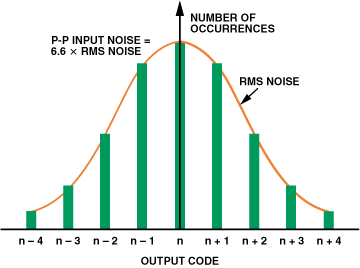Figure 8. Histogram for an ADC measuring a constant analog input.

An averaging filter is a good way to reduce random white noise while keeping the sharpest step response. The software for the design discussed here uses a moving-averaging algorithm. Figure 9 shows the basic algorithm flow.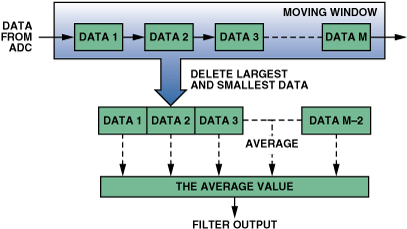Figure 9. Averaging algorithm.

A moving-average filter averages a number of points from the input signal to produce each point in the output signal. The input to the filter is taken directly from the ADC. Operating on the most recent M data points, the smallest and the largest data points (the outliers) are deleted from the data window. The remaining M – 2 points are averaged, as shown in the equation.

Using the moving-average technique, the output data rate remains the same as the input data rate. This is first-order averaging. For higher update rates, second-order averaging is generally used to reduce the waveform dispersion. In that case, the output from the first stage is averaged through a second stage to further improve results.

Figure 10 shows the measured data from the AD7799 after averaging. Comparing this to Figure 5: after averaging there is an improvement of about 2.3 bits in the final result (21.9-bit vs. 19.6-bit effective resolution). This technique can dramatically improve the final result, with no effect on LCD-output update rate. The only disadvantage of this technique is a longer settling time due to the pipeline delay of the averaging.Figure 10. AD7799 noise performance after filtering at: gain = 64, update rate = 4.17 Hz, reference = 5 V, load-cell input. RMS noise = 0.611 LSBs, p-p resolution = 21.9 bits.

#### Improving the Response Time to Weight Changes

The basic algorithm can improve the noise performance, but it has a problem when the weight is changed. After a weight change, the output of the load cell should move to another balanced state in a very short time. According to the algorithm, the output of the filter can only indicate the most correct result after the filter refreshes M times. The response time is limited by the number of averaging points. A specific algorithm is needed to judge the change of the weight. Figure 11 shows the basic flow of this algorithm.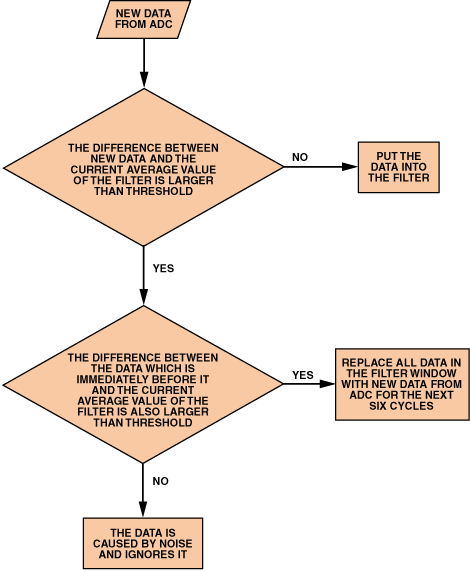Figure 11. Weight-change judgment algorithm.

First, doubled judging steps are used in order to avoid taking a glitch as a weight change. When the differences between two continuous data points from the ADC and the output of the filter both exceed the threshold, this is considered as a weight change.

All M points of the second stage will be filled with the same new data in order to skip the transition period of the load cell very quickly after weight change. Also, the load cell itself has a settling time. To compensate for this, after a weight change is detected, all the data in the averaging moving window will be refreshed with the newest ADC data for the next six continuous averaging cycles to pass the recovery time by. After the six refreshing cycles, the averaging will resume.

#### Removing Flicker on the Output Result

The weigh scale is aligned to display 0.5-gram divisions or 1-gram divisions for 1:5,000 and 1:10,000 standard ranges. When the weight is in the margin between two adjacent display weights, the display will flicker between these weights. In order to keep the display stable, the algorithm in Figure 12 is used:Figure 12. Code-change flow chart.

In every display cycle, the software decides whether the displayed weight in this cycle is the same as the previous one. If it is the same, the LCD output will not change and the process continues to the next cycle. If it is different, the internal code difference between these two cycles will be calculated. If the difference is smaller than the threshold, it is regarded as noise effect, so the old weight will still be displayed. If the difference is bigger than the threshold, it will update the display.

#### Comparing ADuC847 and AD7799 ADC Performance

For low-cost weigh-scale design, the ADuC847, with its on-board ADC, can provide a single-chip solution. The ADuC847 integrates a 24-bit sigma-delta ADC and an 8052 microcontroller core. The internal ADC also has a gain-of-128 PGA with differential analog inputs and reference inputs. It also includes 62K bytes of on-chip program flash memory and 4K bytes of on-chip data flash memory. The following plots, in Figures 13 and 14, compare the integrated ADC on the ADuC847 with the lower noise standalone AD7799. The conditions for both tests are the same: analog inputs are shorted to the 2.5-V reference and a gain of 64 is used. As we would expect, the AD7799 has lower noise and so is suitable for high-end applications, whereas the ADuC847 would be suitable for less demanding weigh scales.Figure 13. AD7799 noise performance: gain = 64, update rate = 4.17 Hz, reference = 5 V, inputs shorted to reference. RMS noise = 3.2526 LSBs, p-p resolution = 19.576 bits.Figure 14. ADuC847 noise performance: gain = 64, update rate = 5.35 Hz, reference = 2.5 V, inputs are shorted to reference. RMS noise = 74.65 LSBs, p-p resolution = 15 bits, data sheet spec = 15 bits.

### Weigh-Scale Design Considerations

#### Ratiometric Design

For best performance, ratiometric measurement techniques (same reference source for bridge excitation and ADC reference) are employed in the reference design, as in Figure 3. The output accuracy of the load cell is determined by the excitation voltage of the bridge. The bridge output is directly proportional to the excitation voltage, and any drift in the excitation voltage produces a corresponding drift in the output voltage. By using a voltage that is proportional to the bridge excitation voltage as the ADC’s reference source, there is no loss in measurement accuracy if the actual bridge excitation voltage varies. This ratiometric connection removes the effect of drifts and very low-frequency noise in the excitation source. In order to filter out noise from the load cell at the inputs to the ADC, a simple first-order RC filter can be used.

#### Layout

Layout is very critical for best noise performance using a high-precision sigma-delta ADC. The most important two aspects are grounding and power-supply decoupling. In this reference design, the ground plane is separated into analog- and digital sections. The AD7799 sits above the split between these two ground planes. One starting point is used to connect the ground planes just under the AD7799. The GND pin of AD7799 should connect to the analog ground. In this design, just one power supply is used, but a ferrite bead goes between AVDD and DVDD terminals. The ferrite bead features low impedance at low frequencies and high impedance at high frequencies. Therefore, the ferrite bead blocks the high frequency noise in DVDD. When selecting a ferrite bead, one should investigate its impedance-vs.-frequency characteristic. In this design, a 600-ohm surface-mount-package ferrite bead is selected. Finally, 0.1μF and 10-μF capacitors are used to decouple AVDD and DVDD supplies; they should be placed as closely as possible to the device.

#### Hardware and Software

The AD7799/ADuC847 weigh-scale reference design can also be interfaced to any PC, using an RS-232 interface. This allows the user to save and process data when evaluating the system. The hardware and software specifications of the reference design are freely available, including source code, schematic and PCB Gerber files. Please contact the authors for more information.